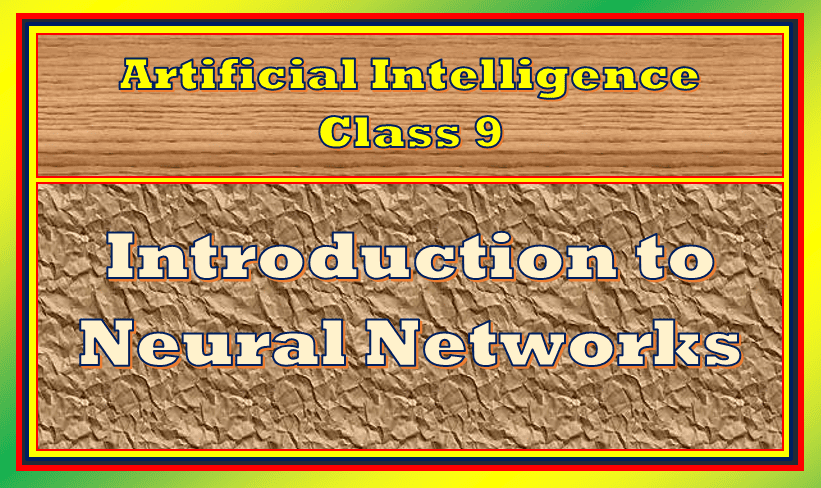We have covered the modeling part in the Unit 2 AI project cycle, now we will start unit 3 Neural Networks for AI Class 9. Let’s start the article now!

Topics Covered

## Introduction to Neural Networks for AI Class 9

The unit 3 neural networks consist of the learning outcome as understand and appreciate the concept of Neural Network through gamification. The session or activity or practical consists of following topics:

1. Relation between the neural network and nervous system in human body
2. Describing the function of neural network

and then we will discuss about an activity given in your syllabus creating human neural network.

So let’s start with introduction to Neural Network for Notes Neural Networks for AI Class 9.

The neural networks are the model that how neurons in the human brain behave. It is also known trained as ANN(Artificial Neural Network) that copy the working of the human brain neurons or cells inside the computers. The greatest thing of ANN is it can extract the features of data without any programming or input. The computer can learn, recognise, and make decisions like human beings.

The neural network works on machine learning algorithms to fulfil the need of the user or perform the task. It basically used to solve the problems for large dataset.

The traditional Machine Learning algorithm cannot improve the performance of the model after certain levels and then saturation will start. Whereas Small ANN performs better than as Medium and Large Neural Network dataset. Observe the following image given in your CBSE handbook:

Now lets understand how neural networks works for Notes Neural Networks for AI Class 9.

## How neural network works?

Observe the image given below:

This image shows the representation of how neural networks work. This image shows that neural network is divided into different layers and each layer is divided into a block that accomplish its own task and then passes to the next layer. The first layer of neural network is known as input layer that acquires the data and feed it to the neural network. Some hidden layers are there which are not visible but all processing occurs in these layers. These hidden layers have its own machine learning algorithm which is executes on the data received from the input layer. Then the processed output is fed to subsequent hidden layer. Similarly, at the last hidden layer passes the final processed data to the output layer.

Now the next section of Notes Neural Networks for AI Class 9 we will talk about features of NN.

## Features of Neural Network

1. NN systems are modelled on the human brain and nervous system
2. It can extract data without any input from the user
3. Every node is essentially a machine learning algorithm
4. Useful when solving problems for which the data set is very large

In the next section of Notes Neural Networks for AI Class 9 we will talk about neural networks vs nervous system.

## Neural Networks Vs Nervous System

As you know computers can perform complex tasks which human beings can’t do in an easy manner. We can’t do complex millions of computations per second. Similarly, computers also can’t do some easy tasks. Here Artificial Intelligence plays a role. ANN creates that are based on the working of the human brain. These networks solve complex computation problems by regulating how the computing cells are activated.

Now in the next section of Notes Neural Networks for AI Class 9 we will see the human brain and its functions in short.

The human brain is made up of billions of interconnected neurons. The interconnections are highly complex. The neurons working in parallel exchanging information through their connectors ‘synapses’. Billions of connections among billions of neurons! Each neuron is talking to millions of other neurons. Each neuron of the brain is made up of a cell body. These cell bodies are tiny. An area of a single square millimetre can contain approximately 100 cell bodies. There are several dendrites attached to the cell body and a single axon.

Now just have a look at how neural networks learn for Notes Neural Networks for AI Class 9.

## How neural networks learn!

There are three ways of neural networks learning:

1. Supervised Learning
2. Unsupervised Learning
3. Reinforcement Learning

### Supervised Learning

As the name supervised learning indicates there is a supervisor like teacher who teaches or trains the model with respect to the output. In this type of learning, networks are trained to provide the correct output by using several example inputs. For example, the network can be trained on the data of students and marks. After training the network will be able to calculate the marks based on various elements like the length of the answer, correct facts written in the answers, comprehension length etc.

### Unsupervised Learning

In this learning no output related information received by the neural network and it provides only input. The system evaluates and find out how different elements are related. This method used for solving clustering problems, estimation problems, self-organizing maps etc.

### Reinforcement Learning

Reinforcement learning, the neural network learn based on learning from experience. Unlike supervised learning, reinforcement learning doesn’t have any output information. It is employed by various software and machines to find the best possible behavior or path it should take in a specific situation.

So in the next article we will do some activities for Notes Neural Networks for AI Class 9. So I hope you understand the concept of neural network. If you have any doubts or queries you can ask in the comment section.